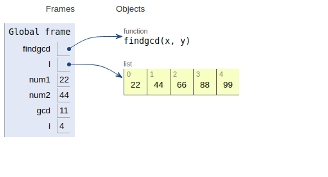# GCD of more than two (or array) numbers in Python Program

PythonServer Side ProgrammingProgramming

## Problem statement

We will be given an array of number and we need to find the greatest common divisor.

If we need to find gcd of more than two numbers, gcd is equal to the product of the prime factors common to all the numbers provided as arguments. It can also be calculated by repeatedly taking the GCDs of pairs of numbers of arguments.

Here we will be implementing the latter approach

So now, let’s see the implementation

## Example

Live Demo

def findgcd(x, y):
while(y):
x, y = y, x % y
return x
l = [22, 44, 66, 88, 99]
num1 = l
num2 = l
gcd = findgcd(num1,num2)
for i in range(2,len(l)):
gcd = findgcd(gcd,l[i])
print("gcd is: ",gcd)

## Output

Gcd is: 11

All the variables and functions are declared in global scope as shown in the image below −## Conclusion

In this article, we learned the approach to find the greatest common divisor of a given array of arguments.